# Prime Numbers to 20

Prime Numbers to 20#### Composite Numbers• Composite numbers can be broken down into two other smaller whole numbers.
• We say that composite numbers are numbers that can be made by multiplying any two whole numbers that are both larger than 1.
• In this example, 4 is broken down into 2 × 2.
• Because 4 can be made by multiplying 2 by 2, we say it is a composite number.
• Not all numbers can be made by multiplying two smaller whole numbers.• Prime numbers are numbers that cannot be broken down into two other smaller numbers.
• Prime numbers can only be written as 1 × themselves.
• The number 2 is the first prime number.
• It can only be written as 1 × 2.
• We say that a prime number has exactly 2 factors, which are just 1 and the number itself.
• 2 is the only even prime number.
• A number is not prime if it is in the times table of another number.

#### List of Prime Numbers to 20The list of prime numbers to 20 is: 2, 3, 5, 7, 11, 13, 17 and 19.

Prime numbers cannot be made by multiplying 2 other smaller whole numbers.

Composite numbers can.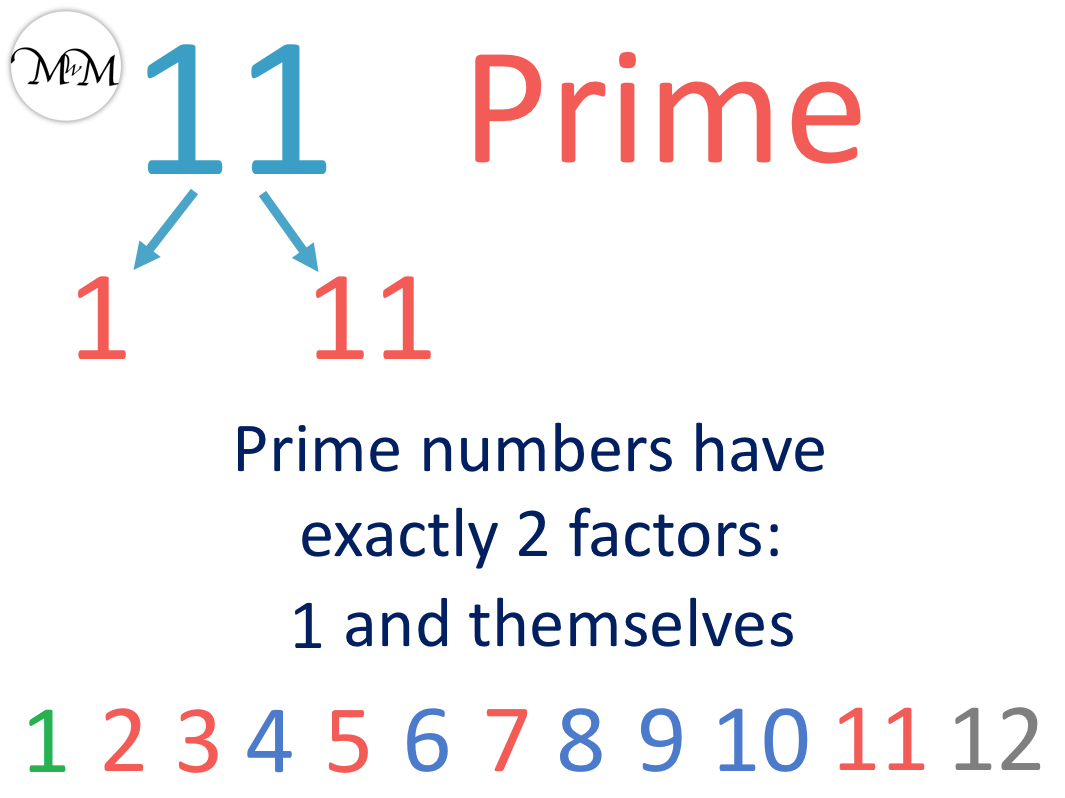• 11 is an example of a prime number.
• 11 cannot be made by multiplying two smaller whole numbers together.
• 11 can only be written as 1 × 11.
• We cannot divide any other whole number into 11 exactly.
• We can try dividing by 2, 3, 4, 5, 6, 7, 8, 9 or 10 and see that they do not go into 11 exactly.Supporting Lessons# Prime and Composite Numbers to 20

## What are Composite Numbers?

A composite number is a number that is made by multiplying two other whole numbers.

For example, 4 is a composite number because it can be made by multiplying two 2’s together.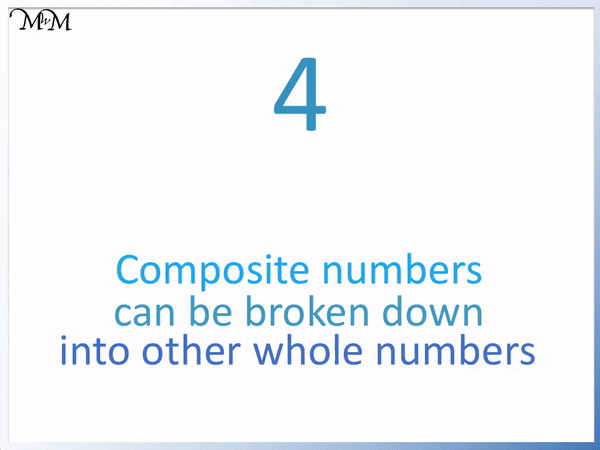Composite numbers can be broken down into two smaller whole numbers. 4 is broken down into two 2’s. 2 is a different number to 4 and it is a smaller number than 4.

To test if a number is a composite number, try to divide smaller whole numbers into it. If a whole number can divide into it exactly, then it is a composite number.

The numbers that divide into a composite number exactly are called factors.

## What are Prime Numbers?

A prime number has exactly 2 different factors, which are the number 1 and the number itself. This means that a prime number cannot be made by multiplying two other whole numbers.

Apart from the number 1 and the number itself, no other whole numbers will divide into a prime number exactly.

2 is the first prime number. It is also the only even prime number.

It can only be made by multiplying 1 × 2.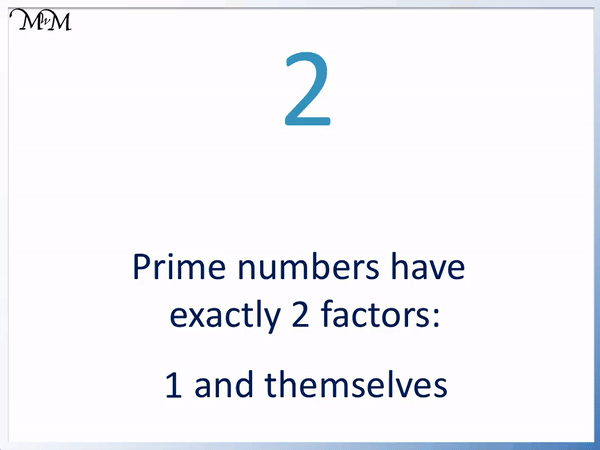There are no other numbers that can divide into 2 exactly.

The only way to make 2 by multiplying is 1 × 2.

When we do this, we still end up with a 2 that is multiplied by 1. It is the same number that we started with and it did not get any smaller.

Because only the number 1 and the number 2 itself can divide into 2, we call it a prime number. We can see it only has two factors, the number 1 and the number 2.

A number is not prime if it is in another number’s times tables.

Prime numbers are hard to find because there is no pattern or rule to find them. We must try and divide all of the smaller whole numbers into a number to test if a number is prime.

## Is 1 a Prime Number?

1 is not a prime number. 1 is also not a composite number. It is neither prime nor composite.

This is because the definition of a prime number is a number that has exactly two factors. These are the whole numbers that divide exactly into a number.

Basically, 1 cannot be broken down into smaller whole numbers.

The number 1 can only be written as 1 × 1.Therefore it only has one factor, which is the number 1. It does not matter that there are two of them, it is still the same number. A number needs exactly 2 different factors to be a prime. 1 does not have enough factors.

To be a composite number, the number needs to be made by multiplying two other smaller whole numbers.

1 is only made by multiplying 1 by 1. This is the same number and it is not smaller. 1 is not a composite number either.

1 is neither prime nor composite.

It is worth pointing out that it is a common mistake to count 1 as a prime number. This is because people think of prime numbers as numbers that cannot be broken down, whereas the proper definition is to check if it has two factors, which the number 1 doesn’t.

Apart from the number 1, all whole numbers are either prime or composite. If they are not prime, then they are composite and if they are not composite, they are prime.

## Finding Prime Numbers from 1 to 12

We will look at the list of numbers to 12 and identify the prime numbers.

1 is neither prime nor composite because it cannot be made by multiplying two smaller whole numbers.All of the other numbers larger than 1 are either prime or composite.

2 is a prime number. It can only be made by multiplying the whole numbers 1 × 2.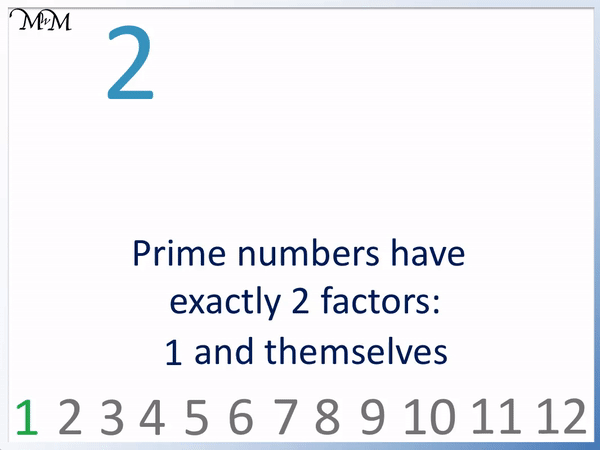2 is special because it is the first prime number. It is also the only even prime number.

This is because any other even number can be divided by 2 as well. A prime number cannot be divided by any number apart from 1 and the number itself.

It is a common mistake to think that 2 is not prime because it is even and it is worth highlighting this number when teaching prime numbers.

The number 3 is also prime.

It can only be written as 1 × 3. So it has 2 factors, 1 and 3.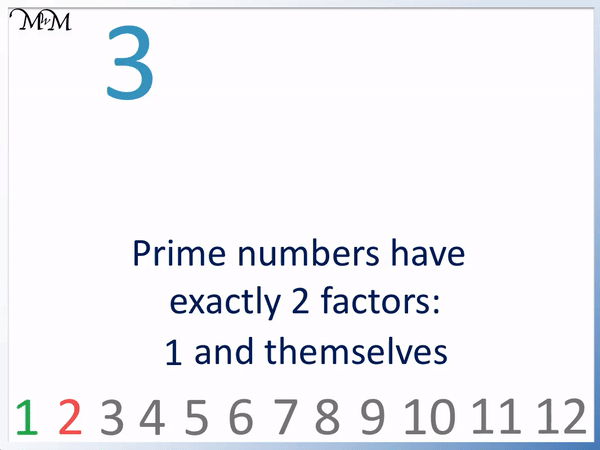The only other smaller number than 3 is 2. We can try dividing 2 into 3 to check, but 2 does not go exactly into 3 because 3 is an odd number.

4 is a composite number made from 2 × 2.

Even though it can still be written as 1 × 4, we have this extra option of 2 × 2.

4 can be broken down into smaller whole numbers.4 is even and all even numbers, apart from the number 2, are prime.

Because 4 is composite it is not prime.

5 is a prime number. It only has 2 factors, 1 and itself. This means that it can only be written as 1 × 5.To check if 5 is prime, we can try dividing other smaller numbers into it.

2 does not go into 5 because 5 is odd.

5 is not in the 3 times table or the 4 times table either.

6 is a composite number.

It is even and so it is in the 2 times table.6 can be made from 2 × 3 and so it is not a prime number.

7 is a prime number.

It can only be made from 1 × 7. It has no other factors.8 is a composite number because it is even.

It can be written as 2 × 4.Because 8 is composite, it is not prime.

9 is a composite number and is not prime.

9 can be written as 3 × 3.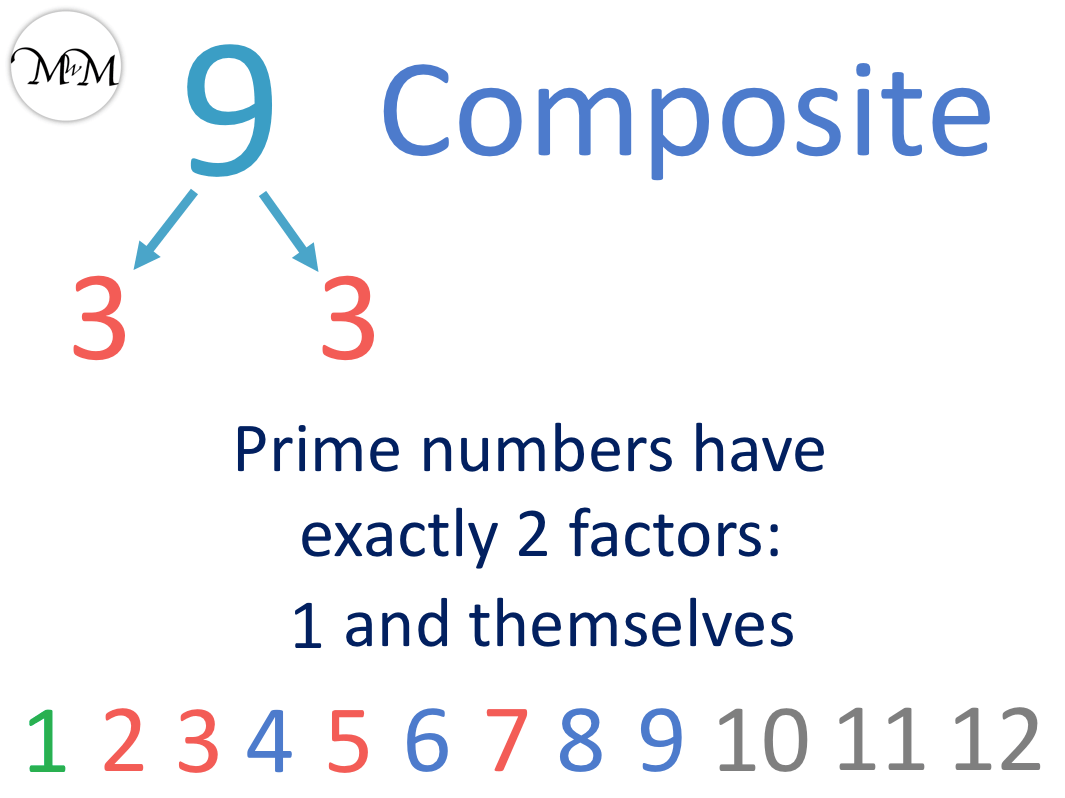It is a common mistake to include 9 as a prime number because it is odd. It is our first odd number that is not prime. 9 is 3 times 3 and so, it can be broken down into smaller whole numbers.

10 is a composite number because it is even.

10 is in the 2 times table and the 5 times table.10 is not a prime number.

11 is a prime number. 11 can only be written as 1 × 11.12 is a composite number.

12 can be written as 1 × 12, 2 × 6 and 3 × 4.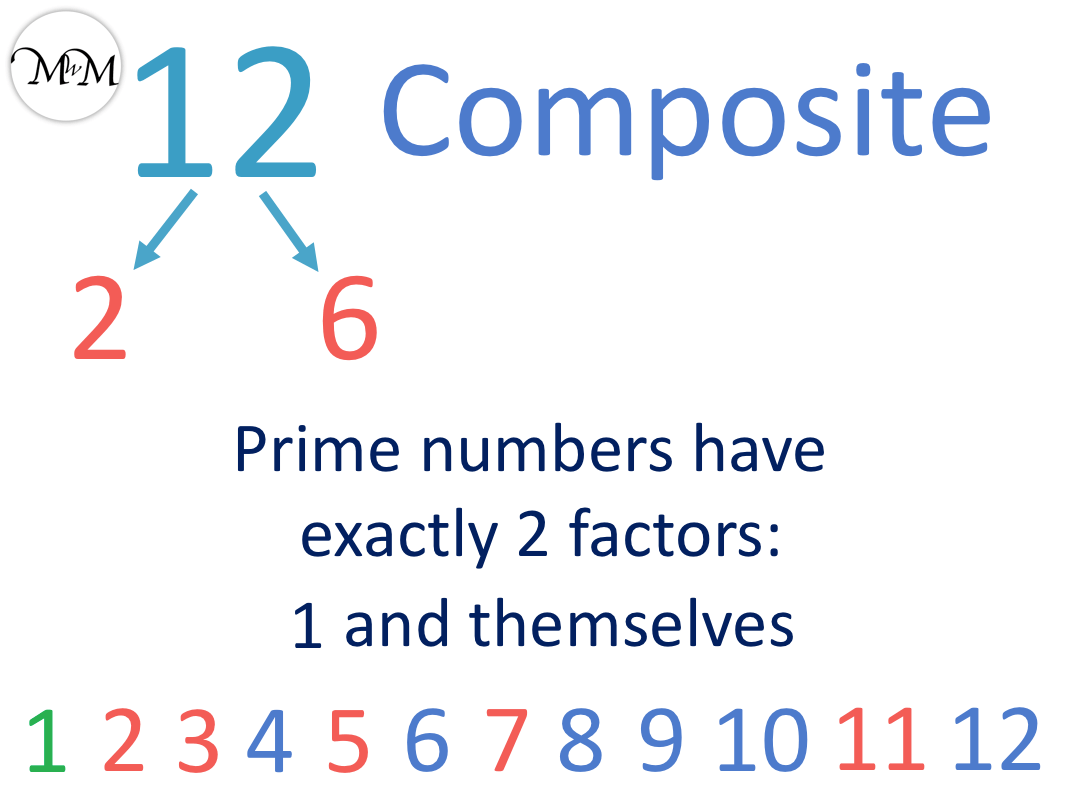12 is not prime.

## Finding Prime Numbers using the Sieve of Eratosthenes

Finding prime numbers is very difficult because there is no real pattern to them.

There is no quick way to find prime numbers. We must check to see if any smaller numbers divide into our number.

One way to find prime numbers is using the Sieve of Eratosthenes.

The Sieve of Eratosthenes is named after a Greek mathematician, Eratosthenes, who it is attributed to.

It uses the fact that a prime number will not be in the times table of any smaller number.

‘Prime’ is another word for ‘first’ and a prime number will be the first number in a times table as long as it does not appear in any other times table.

We start with a number grid and cross off the numbers in each times table.

We know that 1 is neither prime nor composite and so we will mark this in green to show this.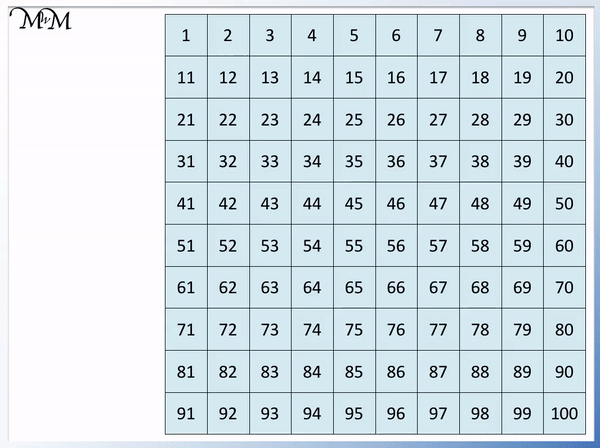We mark the first number in this times table as prime and cross off the numbers in the two times table that come after.

Any number in the two times table that is larger than 2 will not be prime. This is because they can be divided exactly by 2.

Once we have marked the two times table, we look at the next times table.

We mark the first number in the three times table, 3, as prime and then cross off the remaining numbers in the three times table.Some numbers in the 3 times table are already crossed off because they were in the 2 times table but we cross off the rest.

Next is the 4 times table, however all numbers in the 4 times table are also in the two times table.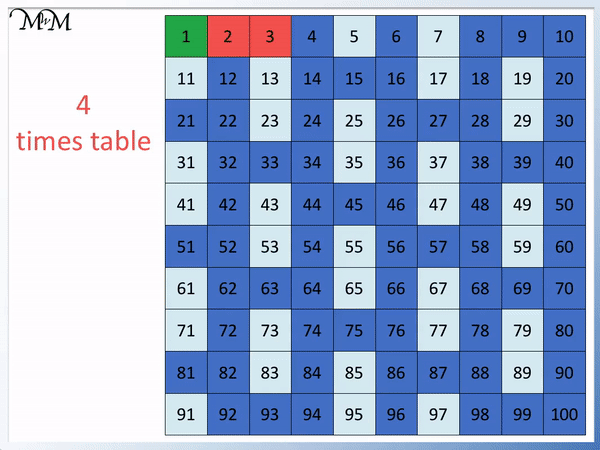Next we mark 5 as prime and then every other number in this times table is marked as composite.All of the numbers in the 6 times table have already been marked.

This is because 6 is in the 2 times table and the 3 times table.We now mark the 7 times table. 7 is prime and we cross the next numbers as composite.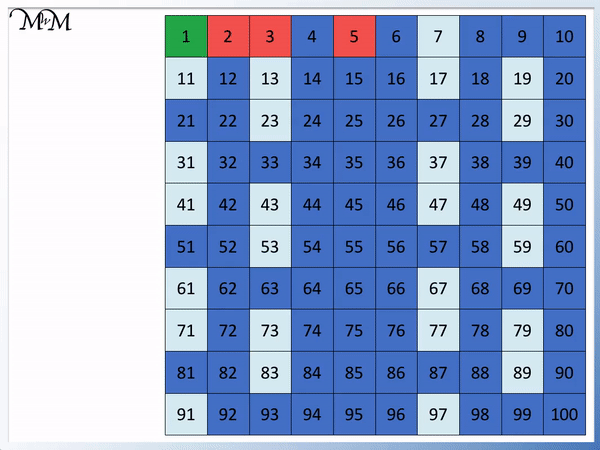The numbers that have been crossed off in blue on this grid are composite numbers.

All of the remaining numbers that are not crossed off on this grid are prime numbers. We will shade them in red.Below are the prime numbers to 20.Now try our lesson on Negative Numbers on a Number Line where we learn about negative numbers.error: Content is protected !!